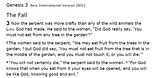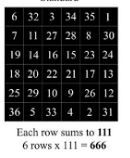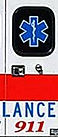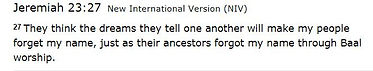## class 19 numbers  of the serpent god of the Moonsee pages  insight into the kabbalah.and thename OF THE serpent"GOD"OF THE OF KABALLAH CONSIDER THIS PAGE AN introduction to an ancient language which is  i kept alive within the Kabbalah and is   a demonic language understood and utilized by the controlling powers of this fallen  world. stay tuned  for a possible presentation with an esoteric reveal of this hidden language. see images. in the kabbalah it is  common to "triangle" a number(calculate the number of characters that would fit in a triangle for  a word or number. see image of
Tet·ra·gram·ma·ton

1. the Hebrew name of their God"" transliterated in four letters as YHWH or JHVH and articulated as Yahweh or Jehovah.

yahweh(yod 10, heh5, vav, 6 heh5, = 26(4 characters =10 within triangle and total 72  note  its 72 to each angle(beliefthat spirits travel in angles (72+72+72=216 (6x6x6=216 see video of pi movie. note 216 is  also  the diameter of the moon moon in miles 2160miles(0 at end does not count Toth is the"god" of the moon.  note6x6=216 ( also a cube(see star of rephan Lucifer page.  and . note a triangle up = fire as in the sun or Baa( so does  a tetrahedron (tetra)see images.(note 6x6x6=216 therefore some of the numbers the   of the serpent= =26,72,2160 AND   9= moon in kABBALLAH YES 9 -11 DIRECTLY LINKS TO THESERPENT(more on 9-11 and its relation to 911.   SEE IMAGE OF MAGIC SQUARE OF THE SUN=666 . NOTE 666/212=BOLING TEMP OF Water(moon represents water = pi 3.14Pic of the tree of good and EVIL not lifeHelvA HEXAGON OR STAR OF REPHAN=720

6 x6x6=216pathway OF HE MIDDLE=THE SERPENT SEE KABBALAH TREE(MORE LATER FOR NOW THE total OF THE Middle path=the Serprent= 26 the totalof tetragrammaton yahweh 1+10+9+6 =26the SerpentYahweh see the "name of God page"Serpent "god"of the Kabbalah. Yod Heh Vav Heh. Yah is made from  the hebrew yod=10, heh=5 total=15, See Yahoo Yah=15, 0=15(15th letter of alphabet so therefore yahoo= 15 15 15. now 15=1+5=6 so YAHOO=666